# Maximum likelihood estimation

## 2003-05-06

Dear Professor Mean, What is maximum likelihood estimation and how does it work?

Maximum likelihood is an approach that looks at a large class of distributions and then chooses the “best” distribution. For each distribution, we compute a likelihood, and the best distribution is the one that maximizes this likelihood.

Suppose we have a set of three data points, and we want to find the normal distribution that is “best” for this data. We would look at a variety of bell-shaped curves, and measure the likelihood for each bell shaped curve. In this case, you compute the likelihood by measuring the height of the bell at each data point and then multiply those heights together. Shown below are three data points: 0.1, 0.4, and 0.8 along with a bell shaped curve.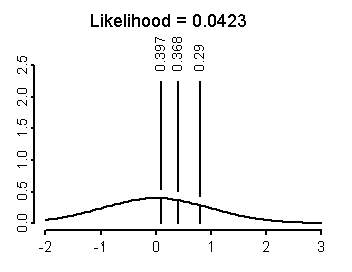This particular bell shaped curve has the values mu=0 and sigma=1. The heights are 0.397, 0.368, and 0.29. When you multiply these values together, you get a likelihood of 0.0423. That’s not bad. Let’s see if we can do better. Shown below is the same data with a new bell shaped curve.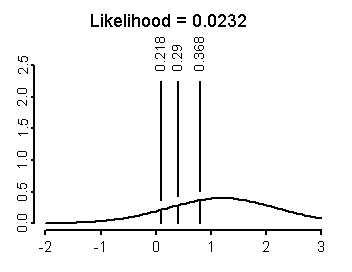Here the bell shaped curve has mu=1.2 and sigma=1. This bell shaped curve is not as good, because the likelihood is only 0.0232. Let’s try a third bell shaped curve.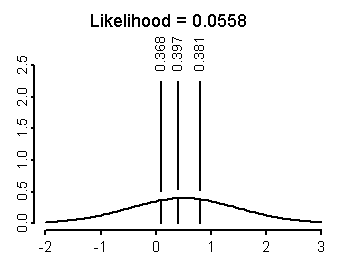In this bell shaped curve, mu=0.5 and sigma=1. This is the best so far, with a likelihood of 0.0588. But we can improve further by reducing the value for sigma.Here, mu=0.5 and sigma=0.4. Notice now that the likelihood is 0.4402, a substantial improvement over the previous three distributions. What happens if we reduce sigma even further?Oops! Too far. In the example above, mu=0.5 and sigma=0.2. The likelihood has decreased to 0.3077.

You could continue by trial and error and eventually you would find that the distribution with mu=0.433 and sigma=0.287 maximizes the likelihood. You could then interpret this particular bell shaped curve as the “best” meaning the most consistent with the data. The estimates 0.433 and 0.287 are then called the maximum likelihood estimates of mu and sigma.

There are better approaches than trial and error. For many situations, a bit of algebra and calculus can help you identify the maximum likelihood estimates. You know, for example, that the formula for the height of a bell shaped curve is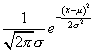so you could substitute the values of 0.1, 0.4, and 0.8 into the above formula and then multiply them all together. You would get something like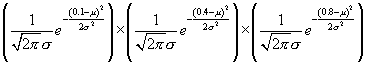and with a bit of patience, you could simplify this formula, take the first derivative with respect to mu and sigma and set those derivatives equal to zero.

You could also work out a solution for a general case where you have a sample of n data points, x1, x2,…,xn. The likelihood would be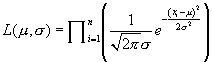Very carefully, you could find derivatives and set these derivatives equal to zero. The solution represents the maximum likelihood estimates for the general case.

You may often find it convenient to work with logarithms. The log of the likelihood function often simplifies many of the calculations, and if you find the maximum of the log likelihood that also has to be the maximum of the likelihood itself. In the example above, the log likelihood isThat formula may not look a lot simpler, but it is easier to compute derivatives for the log likelihood in this and many other situations.

I won’t show all the equations, but the maximum likelihood estimate of mu ends up equaling the sample mean and the maximum likelihood estimate of sigma ends up equaling, not the sample standard deviation exactly, but something very close where you replace n-1 with n in the formula.

Sometimes even calculus fails us, and we have to rely on computer approximations. We know a lot about using computers to maximize complex functions, so this is actually better than it sounds.

You can find an earlier version of this page on my original website.